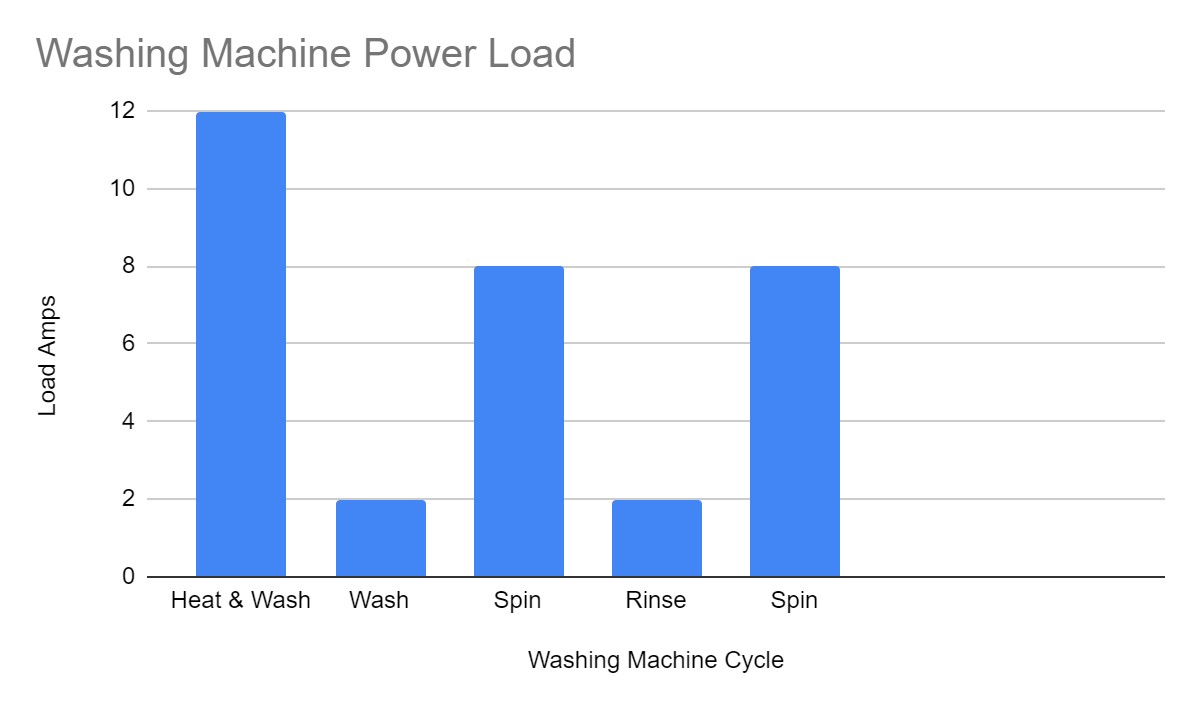# How Do I Calculate Electrical Loads?

Should you leave the calculation for electrical loads to an electrician, or is it something a householder can do easily?

Calculating the electrical load for your home can be tricky, so you should leave it to a professional. You will need to calculate power diversity with the electric loads, which takes some practice. And in certain circumstances, it’s acceptable to use a smaller rated cable for high amperage but with reduced time.

## How do you calculate the electrical load in a house in the UK?

It’s not a simple task, and the calculations can be long, so you should understand what’s needed to develop the final figure for the total electrical load in your home.

Imagine you have three resistors in a simple parallel circuit. The resistors could be a bulb, an appliance or a toaster.

You will need to use the ohms Ω law to find out the amps produced within the circuit to determine the total load.

First, you need to add values such as the voltage flowing in the circuit. You know that 230/240 volts are the voltage, but if you measure at the power outlet, it will be closer to 240v.

Each resistor has a value of 20R1. 80R2 and 60R3 by a simple calculation, you can now find out the current in each resistor and add that to your total load.Alternatively, you can check in the on-site guide, where you can find all the values set out to save you some time. But by using maths, you can find anything you need in the circuit.

## What is the general formula for calculating the total load in kW and kVA?

Calculation of Load in KW :

• 1 phase = V×I×p.f×10^-3
• 3 phase = √3 V×I×p.f × 10^-3

Calculation of Load in KVA :

• 1 phase = V×I×10^-3
• 3 phase = √3 V×I ×10^-3

## How do you account for load diversity?

There are certain applications where you will need to account for load diversity in your calculations. What’s meant by load diversity?

For example, the below graph shows the operation of a washing machine and how it draws current.You can see that during the initial heating and washing phase, the washing machine requires most of the current with the current then dropping off significantly.

## How long does it to do a house load calculation?

This is where experience comes in. An experienced electrician looks at an electric shower and knows what is required. The electrician has a tally of your outlet, light fitting, and possible appliances and can come up with the answer needed within minutes.

## What uses the most electricity in my home UK?

What are the top five energy-consuming home appliances?

• White goods such as washing machines, dishwashers and anything else that uses a water heater in an appliance.
• Tumble dryers use constant high amps
• Cold appliances, your fridge freezer is responsible for using approximately 12% of the average household electricity bill
• Cooking, most ovens are electric and have gas hobs. The electric oven is a large user of electricity
• Consumer electronics
• Lighting

## How many kWh does a UK house use per day?

It depends, but the average is approximately 8.5 to 10 kWh per day. With working couples, the house is empty all day, and then there may be an outdoor sports activity a few nights each week, and out all weekend, so the usage is low in this case.

However, when families come along, the balance swings the other way and the hours spent at home using electricity increases dramatically.

## How do you calculate your electricity bill?

If you want to work out how much electricity an electrical appliance uses in your home, you can use this calculation.

Find the wattage rating of the appliance, such as a TV. It’s generally on a data sticker or in the handbook that comes with the TV under technical specifications.

If your TV is switched on for 5 hours in a day and is rated at 150 W, you have consumed 750 watt-hours in this time.

As your bill marks these things in kilowatts, there are another bit of maths you need to do. To work this out, get your previous total (in this case, 750) and divide it by 1,000 (because 1 kilowatt equals 1,000 watts). So, 750 watt-hours per day/1000 = 0.75 kWh (per day).

If you multiply this number by 30 (the number of days in the month), you will accurately represent what it costs to run this appliance.

On your bill, find out where it says how much they’re charging ‘per kWh’, and multiply it with the monthly figure you worked out before, and you’ll have your total.

## How can I monitor my home electricity usage?

To get down in the weeds, you will need an electric usage monitor to give you the information you want on your appliances and circuits. The electric monitor can be a simple plug load monitor or a more expensive smart home monitor using apps.

The monitors are surprisingly affordable and are purchased online from Amazon.co.uk.

## How much kWh does a house use in the UK?

Ofgem says that the average household in the UK has 2.4 people living in it and uses 8kWh of electricity every day and 33kWh of gas per day.

This works out as an average of 242 kWh of electricity and 1,000 kWh of gas per month, or 2,900 kWh of electricity and 12,000 kWh of gas each year.

## How do you calculate electric loads?

You don’t. Leave it to the professionals. There are too many variables, and you need an experience which will ultimately save you money in the long run.

Electricians spend 4 years training, which is only part of why an electrician’s job can be complex.